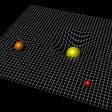# Is There Even Such Thing as Correct Probability?

The more I learn about statistics and probability, the more I realize that even though the mathematical foundations of it are very clear, the arguments that rely on the interpretations of the results provided by statistical analysis of real-world data are generally very vague and questionable.

In this article, I would like to examine how statistical interpretations can be confusing and unclear, and express concern about the fact that the majority of modern science relies on such interpretations.

Statistics — the mathematical study of the likelihood and probability of events occurring based on known information and inferred by taking a limited number of samples. — Wolfram Dictionary

If we were to completely simplify it, we could say that statistics is a way to make arguments about processes where you only know the outcomes, and maybe the initial conditions, but don’t know the path taken. It is a kind of argumentation about black boxes.

For example, in the 52 count card deck when you don’t know the exact order, you can still resolve ambiguity by counting cards, and the more you keep track of the cards, the more certain your guesses become. Obviously, if you knew the exact order of the cards, you wouldn’t need to use probabilities.

Thinking in probabilities is an educated guesswork type of reasoning, useful in situations where a more deterministic way isn’t available.

However, this leads me to the main point. Using this guesswork can be tricky when reasoning about the real world.

Consider the well-known rare disease affecting 1% medical test example. If the test gives the correct results 90% of the time, and your result comes as positive, what is the probability that the result is correct?

I would argue that 90% (chance of getting the correct result) and 1.8% (chance of having a disease given you got the positive result P(D=+|T=+) = P(T=+|D=+)P(D=+)/P(T=+) = 0.9*0.01/0.5 (if we assume 0.45 and 0.05 account for true and false positives) = 0.018) are both correct answers.

I think everybody would agree that if there is 90% chance of getting the correct result, it is just that — 90% chance of getting the correct result, and if you don’t, you essentially won a lottery with odds 1/10, nothing magical.

However, the second example tells us the probability of really having the disease given that you have a positive result, which is a completely different argument because under the hood we are now trying to see how many true positives (part of correct results) there are out of all positives (correct and incorrect results) that we know of, and if the disease is rare, then, of course, there will be way more false positives than true positives in the set of all positives.

Of course, it is harder to win the lottery with the odds of 1/100 than to win the one with the odds of 1/10, which means that if a test for a rare disease came out positive, it is more likely you won an easier lottery.

But I would like to state that both are correct interpretations. We just need to know precisely what these interpretations say. But, unfortunately, it seems too idealistic to expect that the interpreter will always be able to tell the difference. And this is very alarming since in this example the differences between the probabilities are striking.

Is it reasonable to expect that the interpretations would get even trickier when the arguments get more complex (e.g. quantum mechanics)? And if that might be the case, isn’t it alarming that a large part of science is based on statistical arguments?

--

--

--

## More from Kirill Novik

Whether I shall turn out to be a hero of this book these pages must show

Love podcasts or audiobooks? Learn on the go with our new app.

## Solution Insights: Problem on Geometry for 28–01–2022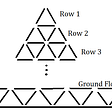## Simulating Kelly Criterion## Math Behind Euclid’s Theorem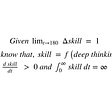## (Deep Bayesian Inference)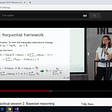## The beauty of mathematics is not obvious…but we can make it so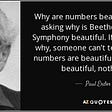## Solving for Eigenstates: The Time-Independent Schrödinger Equation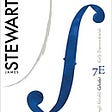## The Old and the New Normal## Kirill Novik

Whether I shall turn out to be a hero of this book these pages must show

## An explanation for Benford’s law## Probability, Statistics and Truth Part 1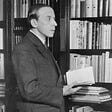## The evolutionary science and mathematics and their ‘miracles’